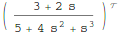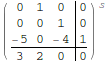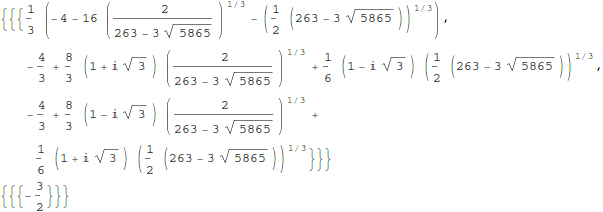## Zero-Pole-Gain models in Mathematica 8

January 17th, 2011 | Categories: mathematica, matlab | Tags:

MATLAB’s control system toolbox has functions for converting transfer functions to either state space representations or zero-pole-gain models as follows

```%Create a simple transfer function in MATLAB
num=[2 3];
den = [1 4 0 5];
mytf=tf(num,den);

%convert this to State Space form
tf2ss(num,den)
ans =

0   1   0
0   0   1
-5  -0  -4

%Convert to zero-pole-gain form
[z,p,k]=tf2zp(num,den)
z = -1.5000
p =

-4.27375 + 0.00000i
0.13687 + 1.07294i
0.13687 - 1.07294i

k =  2```

Now that Mathematica 8 is available I wondered what the Mathematica equivalent to the above would look like. The conversion to a StateSpace model is easy:

```mytf=TransferFunctionModel[(2 s + 3)/(s^3 + 4 s^2 + 5), s]
StateSpaceModel[mytf]```but I couldn’t find an equivalent to MATLAB’s tf2zp function.

I can get the poles and zeros easily enough (as an aside it’s nice that Mathematica can do this exactly)

```TransferFunctionPoles[mytf]
TransferFunctionZeros[mytf]```but what about gain?  There is no TransferFunctionGain[] function as you might expect. There is a TransferFunctionFactor[] function but that doesn’t do what I want either.

It turns out that there is a function that will do what I want but it is hidden from the user a little in an internal function

```Control`ZeroPoleGainModel[mytf]

Control`ZeroPoleGainModel[{{{{-(3/2)}}}, {1/
3 (-4 - 16 (2/(263 - 3 Sqrt))^(
1/3) - (1/2 (263 - 3 Sqrt))^(1/3)), -(4/3) +
8/3 (1 + I Sqrt) (2/(263 - 3 Sqrt))^(1/3) +
1/6 (1 - I Sqrt) (1/2 (263 - 3 Sqrt))^(1/3), -(4/3) +
8/3 (1 - I Sqrt) (2/(263 - 3 Sqrt))^(1/3) +
1/6 (1 + I Sqrt) (1/2 (263 - 3 Sqrt))^(1/3)}, {{2}}}, s]```

That’s not as user friendly as it could be though. How about this?

```tf2zpk[x_] := Module[{z, p, k,zpkmodel},
zpkmodel = Control`ZeroPoleGainModel[x];
z = zpkmodel[[1, 1, 1, 1]];
p = zpkmodel[[1, 2]];
k = zpkmodel[[1, 3, 1]];
{z, p, k}
]```

Now we can get very similar behavior to MATLAB:

```In:= tf2zpk[mytf] // N

Out= {{-1.5}, {-4.27375, 0.136874 + 1.07294 I,
0.136874 - 1.07294 I}, {2.}}```

Hope this helps someone out there.  Thanks to my contact at Wolfram who told me about the Control`ZeroPoleGainModel function.

Other posts you may be interested in